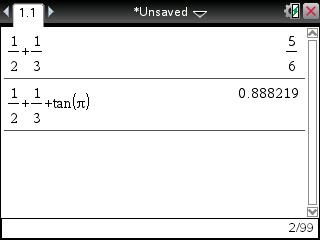# Knowledge Base

## Solution 25890: Exact and Approximate Solutions Using the TI-Nspire™ Handheld and Computer Software.

### Why do I sometimes get a decimal approximation in a stacked fraction calculation on the TI-Nspire family handheld and computer software when I expect to see the answer in stacked fraction form?

Using the TI-Nspire handheld and computer software, if only stacked fractions are used in the input, the resulting answer will also be displayed in stacked fraction form. However, if something is added to the input that approximates to a decimal value, the resulting answer will always be in decimal form.

Example: 1/2 + 1/3 = 5/6

but

1/2 + 1/3 + tan(p) = 0.888219

Please Note: Even though tan(p) = 0, the answer will still be displayed as 0.888219 because the TI-Nspire handheld and computer software approximate the value of tan(p).This approximation feature does not affect the TI-Nspire CAS handheld and computer software. If 1/2 + 1/3 + tan(p) is input using a TI-Nspire CAS handheld or computer software, the resulting answer is 5/6.